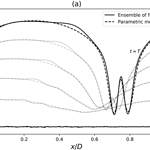https://doi.org/10.5194/npg-25-481-2018
https://doi.org/10.5194/npg-25-481-2018# Parametric covariance dynamics for the nonlinear diffusive Burgers equation

Olivier Pannekoucke, Marc Bocquet, and Richard Ménard

Abstract. The parametric Kalman filter (PKF) is a computationally efficient alternative method to the ensemble Kalman filter. The PKF relies on an approximation of the error covariance matrix by a covariance model with a space–time evolving set of parameters. This study extends the PKF to nonlinear dynamics using the diffusive Burgers equation as an application, focusing on the forecast step of the assimilation cycle. The covariance model considered is based on the diffusion equation, with the diffusion tensor and the error variance as evolving parameters. An analytical derivation of the parameter dynamics highlights a closure issue. Therefore, a closure model is proposed based on the kurtosis of the local correlation functions. Numerical experiments compare the PKF forecast with the statistics obtained from a large ensemble of nonlinear forecasts. These experiments strengthen the closure model and demonstrate the ability of the PKF to reproduce the tangent linear covariance dynamics, at a low numerical cost.

Share